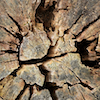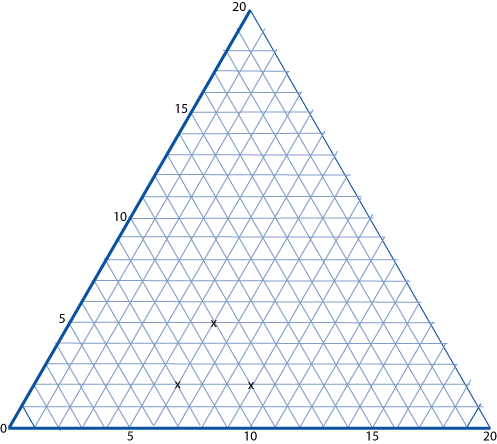#### You may also like### Transformations on a Pegboard

How would you move the bands on the pegboard to alter these shapes?### Timber!

How can the school caretaker be sure that the tree would miss the school buildings if it fell?### Fred the Class Robot

Billy's class had a robot called Fred who could draw with chalk held underneath him. What shapes did the pupils make Fred draw?

# Cartesian Isometric

##### Age 7 to 11Challenge Level

The graph below is a coordinate system based on $60$ $^\circ$ angles. It was drawn on isometric paper.The marked points are $(6, 2)$, $(6, 5)$ and $(9, 2)$. When joined they form an equilateral triangle.

The following five sets of points are also triangles.

A. $(1, 13), (6, 8)$ and $(6, 13)$.
B. $(1, 1), (3, 3)$ and $(7, 1)$.
C. $(12, 1), (17, 1)$ and $(8, 9)$.
D. $(1, 10), (5, 2)$ and $(6, 6)$.
E. $(7, 5), (15, 4)$ and $(7, 11)$.

What kinds of triangles are they?
Can you work out any of the angles at the vertices?